﻿ Evaluation of TIGGE Daily Accumulated Precipitation Forecasts over the Qu River Basin, China
 J. Meteor. Res.2019, Vol. 33Issue (4): 747-764PDF
http://dx.doi.org/10.1007/s13351-019-8096-z
The Chinese Meteorological Society
0

#### Article Information

LIU, Li, Chao GAO, Qian ZHU, et al., 2019.
Evaluation of TIGGE Daily Accumulated Precipitation Forecasts over the Qu River Basin, China. 2019.
J. Meteor. Res., 33(4): 747-764
http://dx.doi.org/10.1007/s13351-019-8096-z

### Article History

in final form April 10, 2019
Evaluation of TIGGE Daily Accumulated Precipitation Forecasts over the Qu River Basin, China
Li LIU1, Chao GAO1, Qian ZHU2, Yue-Ping XU11. Institute of Hydrology and Water Resources, College of Civil Engineering and Architecture, Zhejiang University, Hangzhou 310058;
2. School of Civil Engineering, Southeast University, Nanjing 210096
ABSTRACT: Quantitative precipitation forecasts (QPFs) provided by three operational global ensemble prediction systems (EPSs) from the THORPEX (The Observing System Research and Predictability Experiment) Interactive Grand Global Ensemble (TIGGE) archive were evaluated over the Qu River basin, China during the plum rain and typhoon seasons of 2009–13. Two post-processing methods, the ensemble model output statistics based on censored shifted gamma distribution (CSGD-EMOS) and quantile mapping (QM), were used to reduce bias and to improve the QPFs. The results were evaluated by using three incremental precipitation thresholds and multiple verification metrics. It is demonstrated that QPFs from NCEP and ECMWF presented similarly skillful forecasts, although the ECMWF QPFs performed more satisfactorily in the typhoon season and the NCEP QPFs were better in the plum rain season. Most of the verification metrics showed evident seasonal discriminations, with more satisfactory behavior in the plum rain season. Lighter precipitation tended to be overestimated, but heavier precipitation was always underestimated. The post-processed QPFs showed a significant improvement from the raw forecasts and the effects of post-processing varied with the lead time, precipitation threshold, and EPS. Precipitation was better corrected at longer lead times and higher thresholds. CSGD-EMOS was more effective for probabilistic metrics and the root-mean-square error. QM had a greater effect on removing bias according to bias and categorical metrics, but was unable to warrant reliabilities. In general, raw forecasts can provide acceptable QPFs eight days in advance. After post-processing, the useful forecasts can be significantly extended beyond 10 days, showing promising prospects for flood forecasting.
Key words: TIGGE     quantitative precipitation forecasts     quantile mapping     censored shifted gamma distribution
1 Introduction

Southeast China is prone to flooding in the rainy seasons. From late spring to early summer (the plum rain season), rain belts linger and give rise to prolonged periods of rainfall. The plum rain season ends in early or mid-July and the typhoon season follows with frequent and high-intensity rainstorms. As a result of the complex terrain, there is a high chance that these rainstorms will lead to serious flood disasters (Morss, 2010), with large losses of life and damage to property (Shi et al., 2015). Studies by the Multihazard Mitigation Council (MMC, 2005) and the United Nations Development Programme (UNDP, 2012) have found that it is more cost-effective to invest in emergency responses than in recovery efforts. Hence, improved flood forecasting with a sufficient lead time would benefit disaster preparedness and mitigation (Yang et al., 2015; Liu et al., 2017).

An effective early warning and forecasting system for river floods depends on the timeliness and accuracy of streamflow predictions. An accurate prediction of precipitation is crucially important in accurately predicting streamflow in hydrological models (Gourley and Vieux, 2005; Oudin et al., 2006; Welles et al., 2007; Cuo et al., 2011). An accurate estimate of rainfall at a suitable spatiotemporal resolution is therefore a precondition of accurate flood forecasting (Demargne et al., 2014). However, as a result of the nonlinearity and complexity of atmospheric systems, quantitative precipitation estimates and quantitative precipitation forecasts (QPFs) are still the greatest challenge in weather forecasting (Ganguly and Bras, 2003) and the dominant source of uncertainty in forecasts of streamflow (Cuo et al., 2011).

Historically, ground observation networks (rain gages and remote sensing) were the main sources of precipitation measurements for hydrological, hydrometeorological, and climatological applications. However, radar and/or rain gage measurement networks are inadequate or even nonexistent in many regions of the world (Tong et al., 2013; Miao et al., 2015; Rana et al., 2015; Sarachi et al., 2015; Yucel et al., 2015). In addition, the data can only provide short-term flood forecasts with a lead time of 1–24 h, insufficient for extended flood warnings. The development and improvement of meteorological models in recent years (Gevorgyan, 2013) have accelerated the utilization of QPFs derived from ensembles of numerical weather prediction (NWP) models—known as ensemble prediction systems (EPSs)—to determine the uncertainties in weather forecasts (Cloke and Pappenberger, 2009). These are now routinely used in hydrological and meteorological studies and practice (Khan et al., 2015).

The THORPEX Interactive Grand Global Ensemble (TIGGE), one of the most widely used datasets, was established as the key component of The Observing System Research and Predictability Experiment (THORPEX) project to improve the accuracy of 1-day to 2-week high-impact weather forecasts (Richardson et al., 2005). The techniques for producing these EPS forecasts are based on Monte Carlo simulations. The realization starting from an “unperturbed” analysis is referred to as the control forecast. Other forecasts are generated by the perturbations of the initial condition (the perturbed forecasts) (Cloke and Pappenberger, 2009). More detailed information about TIGGE is reported in Louvet et al. (2016).

Numerous studies have compared the performance of TIGGE EPSs from the point of view of either meteorology or hydrology. Cloke and Pappenberger (2009) comprehensively reviewed successful ensemble flood forecasts using EPSs prior to 2009. Studies have shown that the TIGGE database can provide desirable flood forecasting up to 9 or 10 days ahead for medium to large rivers (Renner et al., 2009; He et al., 2010; Alfieri et al., 2014). Matsueda and Nakazawa (2015) used ensemble forecasts from TIGGE to predict severe weather and found that the grand ensemble provided more reliable forecasts than single-center ensemble. Ye et al. (2014) found that the performance of the ECMWF EPS varies with the properties of the sub-basins. Louvet et al. (2016) assessed QPFs from TIGGE over West Africa and showed that the United Kingdom Met Office and the ECMWF were the best EPSs.

Despite the improvement in the quality of forecast products, forecasts from EPSs generally contain system errors in the mean, spread, and higher moments of their forecast distributions (Verkade et al., 2013), indicating that post-processing is still a requisite to ensure unbiased and properly dispersed forecasts (Madadgar et al., 2014). Numerous methods have been proposed to address this problem. Two of the most common methods are merging single-model forecasts into multi-model forecasts (Bougeault et al., 2010) and using statistical post-processing to calibrate single-model forecasts. An example for the former method is that of Hagedorn et al. (2012), who compared TIGGE multi-model forecasts with reforecast-calibrated ECMWF ensemble forecasts in extratropical regions, concluding that the ECMWF EPS was the main contributor to improved multi-model forecasts. Various post-processing methods have been developed to improve NWP forecasts, such as the extended logistic regression approach (Hamill, 2012), quantile-to-quantile transform (Verkade et al., 2013) and model output statistics (Glahn and Lowry, 1972). More information about post-processing methods can be found in reviews by van Andel et al. (2013) and Li et al. (2017).

This study attempts to provide a detailed evaluation of raw and post-processed QPSs and the degree of improvement in different post-processing methods under different conditions to verify the skill of EPS forecasts in southeastern China during the flood season. Two statistical post-processing methods, the censored shifted gamma distribution based ensemble model output statistics (CSGD-EMOS) and quantile mapping (QM), are selected to post-process forecasts provided by three EPSs. Given the meteorological characteristics of the study area, the assessment is carried out in two separate seasons, namely, the plum rain season and the typhoon season, which is unprecedented in previous studies. The performance is simultaneously evaluated against three incremental rainfall thresholds, differing from previous investigations, which focused only on heavy rain events (Schumacher and Davis, 2010; Wiegand et al., 2011). The paper is constructed as follows. A description of the study area and data used is given in Section 2. Section 3 presents the post-processing and verification methods. The results are provided in Section 4, and the conclusions and discussion follow in Section 5.

2 Study area and data

The Qu River basin, a tributary in the Qiantang River basin, is located in Southwest Zhejiang Province in eastern China and has a catchment area of about 5424 km2. The basin is dominated by a humid subtropical monsoon climate with a total annual mean precipitation of 1500 mm. There are distinct plum rain and typhoon seasons in the wet period. According to Feng and Hong (2007), the plum rain season occurs from May to June and the typhoon season from July to September. Figure 1 shows the location of the Qu River basin and the distribution of meteorological stations.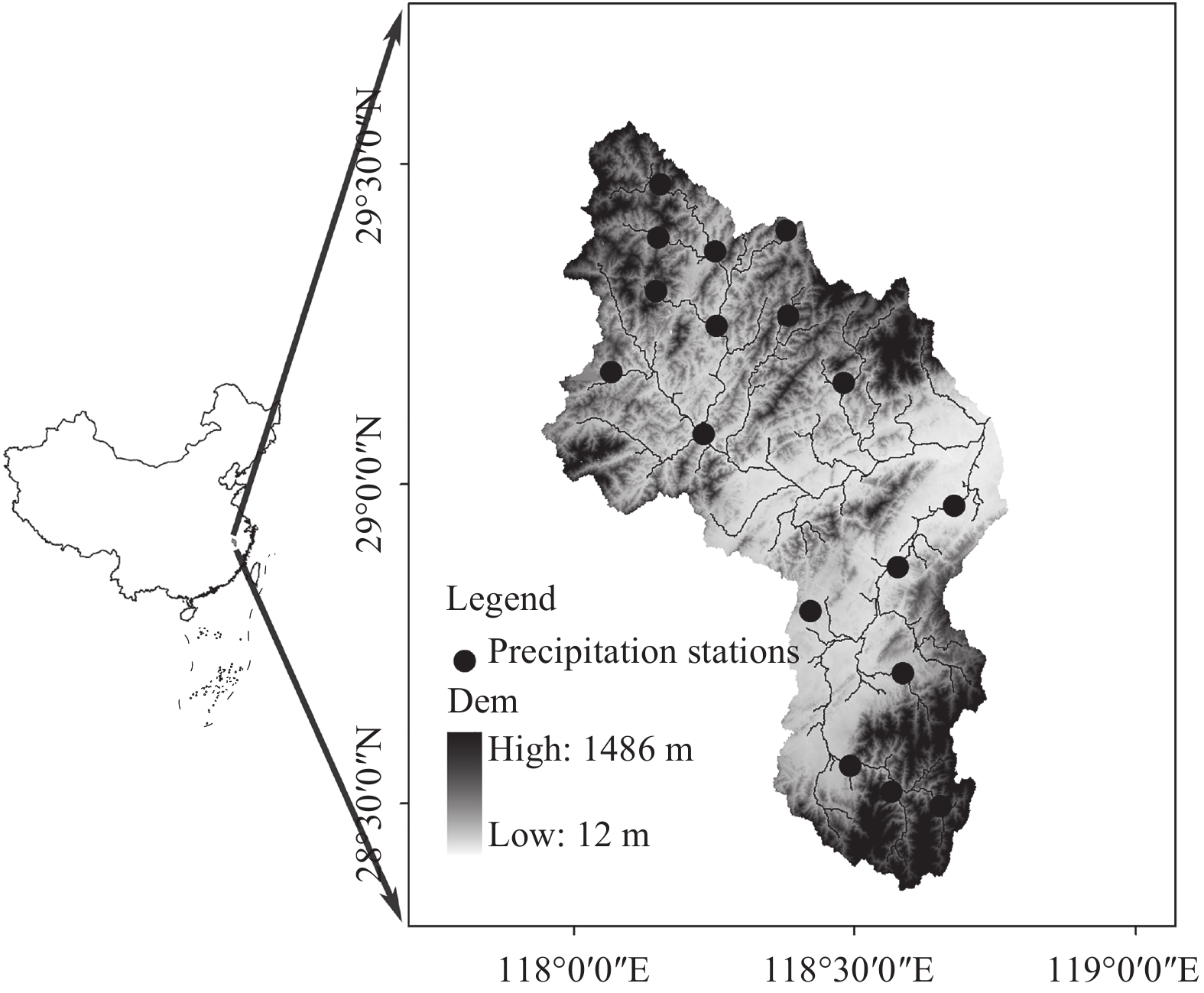Figure 1 Location of the Qu River basin and the distribution of meteorological stations.

Daily observed areal rainfall data were obtained from 17 meteorological stations in the Qu River basin using the Thiessen polygon method. The precipitation is characterized by more frequent, uniformly distributed and heavier precipitation in the plum rain season and more intermittent precipitation in the typhoon season. We use the 24-h accumulated precipitation data forecast at 0000 UTC from three global EPSs, namely the China Meteorological Administration (CMA), the ECMWF and NCEP EPSs, archived in the TIGGE dataset at the ECMWF portal. Details of the three EPSs are given in Table 1 and further information can be found at www.ecmwf.int/.

Table 1 Information about the three TIGGE EPSs investigated in this study
 Center Horizontal resolution in archive No. of ensemble members Lead time (day) CMA 0.56° × 0.56° 14 + 1* 10 ECMWF ~0.28° 50 + 1* 1–10 ~0.56° 11–15 NCEP 1° × 1° 20 + 1* 16 *Control member in each EPS.

All the members (including both the perturbed and control members) of each EPS are used to compute the ensemble-average precipitation with an equal weight combination. The QPF data are downloaded at the original resolution. For convenience of comparison with the corresponding verification observations, we take each grid as a rain gage station and use the Thiessen polygon method to convert the gridded forecasts into areal data. The time period of assessment covers wet periods from 2009 to 2013. Following the World Meteorological Organization standard (WMO, 2012), three thresholds are selected, namely 1, 10, and 20 mm (24 h)−1, representing light, moderate, and heavy rain events, respectively. The highest threshold is the 80% quantile in the plum rain season and the 90% quantile in the typhoon season.

3 Methodology 3.1 Post-processing methods

Two methods are used to post-process the forecast precipitation: CSGD-EMOS and QM. EMOS is a widely used technique in weather forecasting in which raw ensemble forecasts are transformed into a predictive probability density function. It is able to simultaneously correct biases and dispersion errors (Schuhen et al., 2012). The EMOS method has been successfully applied for temperature (Kann et al., 2009) and surface pressure (Gneiting et al., 2005), in which the predictive probability density is a normal distribution. The most popular probability density function for precipitation is the gamma distribution (e.g., Husak et al., 2007; Wright et al. 2017; Elkollaly et al., 2018). However, the gamma distribution has the disadvantage of being unable to fit data with a zero value. To solve this problem, Scheuerer and Hamill (2015) established a CSGD to fit precipitation and a sophisticated heteroscedastic regression model to link observations and forecasts. The cumulative distribution function (CDF) ${\tilde F_{k,\theta,\delta }}$ of the proposed CSGD is defined by:

 ${\tilde F_{k,\theta,\delta }}\left(y \right) = \left\{ {\begin{array}{*{20}{l}} {{F_k}\left({\dfrac{{y - \delta }}{\theta }} \right)}&{{\rm{for}}\;y > 0,}\\ 0&{{\rm{for}}\;y = 0,} \end{array}} \right.$ (1)

where y is precipitation, θ is the scale parameter, Fk denotes the CDF of the gamma distribution with a unit scale parameter and a shape parameter k, and δ is a parameter shifting the CDF of the gamma distribution to the left. The parameters k and θ are the mean (μ) and standard deviation (σ) of the gamma distribution determined by Eqs. (2) and (3):

 $\theta = \dfrac{{{\mu ^2}}}{{{\sigma ^2}}},$ (2)
 $k = \dfrac{{{\sigma ^2}}}{\mu }.$ (3)

The regression model fits the conditional distribution of the observed precipitation given the ensemble forecasts based on the climatological distribution. The climatological distribution is obtained by fitting the parametric gamma CDF ${\tilde F_{{\mu _{cl}},{\sigma _{cl}},{\delta _{cl}}}}$ to the empirical CDF (ECDF) of the observations. The conditional distribution ${\tilde F_{{\mu _f},{\sigma _f},{\delta _f}}}$ is expressed as a function of ${\mu _{cl}},{\sigma _{cl}}$ , and ${\delta _{cl}}$ :

 ${\mu _f} = \dfrac{{{\mu _{cl}}}}{{{\alpha _1}}}{\rm{log}}1{\rm{p}}\left[{\left({{\rm{exp}}\left({{\alpha _1}} \right) - 1} \right)\left({{\alpha _2} + {\alpha _3}{\rm{POP}} + {\alpha _4}{\rm{ENM}}} \right)} \right],$ (4)
 $\hspace{-112pt}{\sigma _f} = {\alpha _5}{\sigma _{cl}}\sqrt {{\mu _f}/{\mu _{cl}}} + {\alpha _6}{\rm{MD}},$ (5)
 $\hspace{-112pt}{\delta _f} = {\delta _{cl}},\quad\quad\quad\quad\quad\;\;\quad\quad\quad$ (6)

in which ${\rm{log}}1{\rm{p}}\left({\rm{x}} \right) = \log \left({1 + x} \right)$ . The parameters ${\alpha _1},{\alpha _2},{\alpha _3},$ ${\alpha _4},{\alpha _5},{\rm and}\;{\alpha _6}$ are calibrated by minimizing the distance between ${\tilde F_{{\mu _f},{\sigma _f},{\delta _f}}}$ and the observations using the shuffled complex evolution algorithm (Duan et al., 1994). POP indicates the probability of precipitation derived from the ensemble, ENM is the forecast ensemble mean, and MD is the dispersion of the forecasts.

QM, also called quantile-to-quantile transform, emanates from the empirical transformation and is widely and successfully used in hydrological applications for downscaling and bias correction (Themeßl et al., 2011, 2012; Zhang et al., 2015). QM focuses on correcting errors in the shape of the distribution based on the ECDF. Figure 2 shows a schematic diagram of the QM correction approach.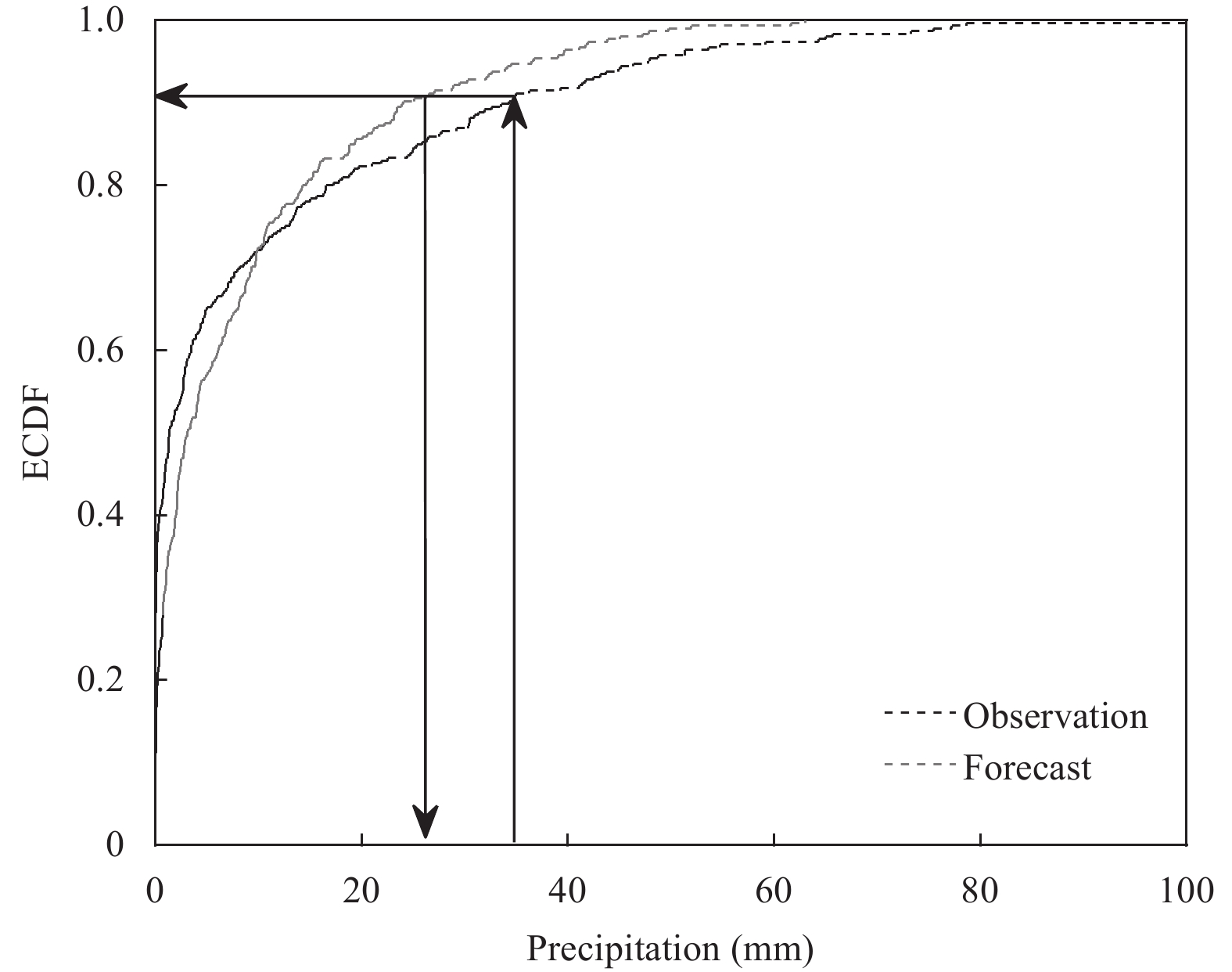Figure 2 Schematic diagram of the quantile mapping correction approach.

This investigation used three steps in the correction of daily precipitation (Themeßl et al., 2012). First, the ECDF(Pr) of the daily raw forecasts obtained from each member of the EPS at each lead time is established by:

 ${\rm{Pr}}{_t} = {\rm{ECDF}}\left({X_t^{\rm{raw}}} \right).$ (7)

The difference (correction function, CF) between the observed and forecasted inverse ECDF (ECDF−1) at probability Pr is then calculated as follow:

 ${\rm{CF}}{_t} = {\rm{ECDF}}{^{\rm{ob{s^{ - 1}}}}}\left({{{{\rm{Pr}} }_t}} \right) - {\rm{ECDF}}{^{\rm{ra{w^{ - 1}}}}}\left({{{{\rm{Pr}} }_t}} \right).$ (8)

A corrected time series Xcor is then obtained:

 $X_t^{\rm{cor}} = X_t^{\rm{raw}} + {\rm{CF}}{_t}.$ (9)
3.2 Verification methods

Multiple statistical verification metrics are used to assess the quality of the QPFs. The Bias and root-mean-square error (RMSE) are representatives of the evaluation of the amount of precipitation. Categorical metrics of the ensemble mean are used to describe the performance in deterministic forecasting. The Brier skill score (BSS) and reliability diagram are used to show the performance of the EPSs with respect to probabilistic forecasting. The continuous ranked probability skill score (CRPSS) is used as an overall evaluation metric.

3.2.1 Bias and RMSE

The Bias and RMSE are the most widely used metrics and are defined as follows:

 ${\rm{Bias}} = \frac{1}{N}\mathop \sum \nolimits_{i = 1}^n \left({{F_i} - {O_i}} \right), \quad\quad\;\;$ (10)
 ${\rm{RMSE}} = \sqrt {\frac{1}{N}\mathop \sum \nolimits_{i = 1}^n {{\left({{F_i} - {O_i}} \right)}^2}},$ (11)

in which Fi and Oi denote the forecast and observed precipitation and N is the sample size. A perfect forecast has Bias = 0. Forecasts with Bias > 0 are overestimated and are underestimated when Bias < 0. The RMSE represents the sample standard deviation of differences between the forecast and observed precipitation in the range 0 to infinity. A smaller RMSE represents a better forecast. For comparison, the Bias and RMSE metrics are calculated for the ensemble mean by assigning an equal weight to each member in the EPSs. For CSDG-EMOS, 5000 samples are randomly sampled from the conditional distribution ${\tilde F_{{\mu _f},{\sigma _f},{\delta _f}}}$ to obtain the ensemble mean.

3.2.2 Categorical metrics

Many meteorological phenomena can be regarded as simple binary (dichotomous “yes/no”) events and forecasts for these events are often issued as categorical statements that they will or will not take place. We consider forecasts as “yes” if the rainfall exceeds a certain threshold. To verify binary forecasts, it is convenient to compile a 2 × 2 contingency table showing the frequency of “yes/no” forecasts and the corresponding observations (Table 2). By definition, either a “hit” or a “correct rejection” is a correct forecast. A wide range of complicated verification metrics can be computed on this seemingly simple table. Nurmi (2003) recommended that in no case is it sufficient to apply only a single verification measure. Five deterministic metrics are used in this study (Table 3). More information can be found in the reviews of Jolliffe and Stephenson (2003) and Wilks (2006). We considered the three thresholds in Section 2 to define a precipitation event as “yes” or “no” and all the metrics are computed based on the ensemble mean of the QPFs.

Table 2 Four possible outcomes for categorical forecasts of a binary event
 Event forecast Event observation Yes No Yes Hit (h) False alarm (f) No Miss (m) Correct rejection (d)
Table 3 Description of categorical verification metrics used in this study
 Verification measure Formula Description Perfect/ no skill Remark Frequency bias index (FBI) ${\rm{FBI}} = \dfrac{{h + f}}{{h + m}}$ Ratio of the number of forecast occurrences to the number of actual occurrences 1/ ≠ 1 FBI = 1, unbiased forecast FBI > 1, overestimation FBI < 1, underestimation Hit rate (HR) ${\rm{HR}} = \dfrac{h}{{h + m}}$ Proportion of occurrences that were correctly forecast 1/0 False alarm ratio (FAR) ${\rm{FAR}} = \dfrac{f}{{h + f}}$ Measure of false alarms given the event did not occur 0/1 Examined with HR (Nurmi, 2003) Critical success index (CSI) ${\rm{CSI}} = \dfrac{h}{{h + f + m}}$ Takes both “miss” and “false alarm” into account, but ignores the “correct rejection” 1/0 Equitable threat score (ETS) ${\rm{ETS}} = \dfrac{{h - r}}{{h + f + m - r}}$ Adjustment of CSI excluding the correct forecast of occurrence by chance 1/ ≤ 0 $r = \dfrac{{\left({h + f} \right) \times \left({h + m} \right)}}{{h + f + m + d}}$
3.2.3 Probabilistic metrics

Categorical metrics often give the forecast two values—0 for “no” or 1 for “yes”—but, in reality, precipitation forecasts become highly uncertain after several days as a result of modeling limitations and the chaos of the earth’s atmosphere (Fundel and Zappa, 2011; Demirel et al., 2013). Therefore, it is incorrect to use only categorical metrics to evaluate forecasts. Probabilistic forecasts attempt to quantify the uncertainty by assigning the event a value between 0 and 100%. We estimated the probability of precipitation as the number of rainy events divided by the total number of events. Based on the probabilistic expression of ensemble forecasts, several probabilistic metrics are designed to verify the EPS forecasts. Two of the most commonly used metrics, the BSS and the reliability diagram, are used here.

The Brier score (BS) was first proposed by Brier (1950) and later improved by other researchers. The commonly used BS is defined by Ferro (2007) and calculated by the following equation:

 ${\rm{BS}} = \frac{1}{N}\mathop \sum \nolimits_{t = 1}^n {\left({{Y_t} - {X_t}} \right)^2},$ (12)

where Yt is the forecast probability of a given event and Xt is the corresponding observed value. For each time t, Xt = 1 if the event occurs and Xt = 0 if not. The score essentially measures the average squared differences between the forecast probabilities and the paired binary observations. The BS is often difficult to interpret. Consequently, the BS is conventionally normalized by a reference forecast (BSref) to convert to a skill score (BSS) (Hamill and Juras, 2006). The relevant climatological relative frequencies are usually used as the reference forecasts (Wilson, 2000). A perfect forecast has BSS = 1. A BSS ≤ 0 indicates no forecast skill compared with the reference forecasts.

 ${\rm{BSS}} = 1 - \frac{{{\rm{BS}}}}{{{\rm{B}}{{\rm{S}}_{{\rm{ref}}}}}}.$ (13)

The reliability of the ensemble forecast is usually indicated by a reliability diagram, which gives a graphical assessment of both the reliability and the resolution of probabilistic forecasts (Shukla et al., 2016; Liu et al., 2017). The correspondence of forecast probabilities with the observed frequency of occurrence is displayed in the diagram. Perfect reliability should lie along the diagonal. Overestimated forecasts appear as curve lying below the diagonal. A flatter curve indicates a lower resolution of the forecasts. We prepared the reliability diagram by partitioning the forecast probabilities into five bins of size 0.2 and estimated the observed frequency for each bin.

3.2.4 Overall metrics

The CRPSS score is calculated based on the continuous ranked probability score (CRPS; Matheson and Winkler, 1976; Hersbach, 2000). The CRPS quantifies the degree of agreement between the cumulative probability distribution of the ensemble forecasts for all the ranges of possible values (no predefined threshold is considered) with the matching single observations (Ye et al., 2014). The CRPS and CRPSS are defined as:

 ${\rm{CRPS}} = \frac{1}{N}\mathop \sum \nolimits_{i = 1}^N \mathop \int \nolimits_{ - \infty }^{ + \infty } {\left[ {F\left(x \right) - H\left({x - {x_{\rm a}}} \right)} \right]^2}{\rm{d}}x,$ (14)
 ${\rm{CRPSS}} = 1 - \frac{{{\rm{CRP}}{{\rm{S}}_{\rm{M}}}}}{{{\rm{CRP}}{{\rm{S}}_{{\rm{ref}}}}}}, \quad\quad\quad\quad\quad\quad\!\!\!\quad\quad\quad$ (15)

in which N is the number of forecasts, x is the forecast variable, and xa is the observed value; F is the distribution function of x and H is the well-known Heaviside function (set to 0 when (xxa) < 0 and 1, otherwise). In this study, the CRPS from raw QPFs in each season is used on behalf of the reference scores for the corresponding season. Thus, the CRPSS herein represents the relative skill score compared with the raw forecasts. A positive CRPSS indicates a positive improvement from post-processing, whereas there is no improvement compared with the raw forecasts if CRPSS is 0 or < 0 ( Daoud et al., 2016). For convenience, the method proposed by Hersbach (2000) is adopted to compute the CRPS, although there is a specific solution for the gamma distribution in Scheuerer and Hamill (2015).

4 Results 4.1 Bias and RMSE

Figures 3 and 4 show Bias and RMSE computed from ensemble mean of the QPFs for three EPSs in both seasons. In the plum rain season (Fig. 3a), all the Bias for the raw forecasts fluctuates along the zero line. Bias of the CMA results is the largest, with lines dramatically deviated from the “Bias = 0” baseline. The QPFs of the CMA show a clear positive Bias for all lead times. The NCEP and ECMWF forecasts provide similarly underestimated QPFs, with Bias ranging from −2 to 0. There are unexpected values for the ECMWF results for the lead time of day + 11, perhaps caused by the different resolutions in the first 10 days (approximately 0.28°) from the last 5 days (approximately 0.56°). The 11th 24-h daily precipitation is obtained from the accumulations of 0–264 h minus those of 0–240 h. In the typhoon season (Fig. 3d), the QPFs of the ECMWF results have an average Bias confined between −1 and 1 mm, whereas the NCEP results have Bias near 2 mm in the plum rain season. There are unexpected values for the ECMWF results in the typhoon season as a result of the same differences in resolution and this fact will not be repeatedly explained in the following discussion.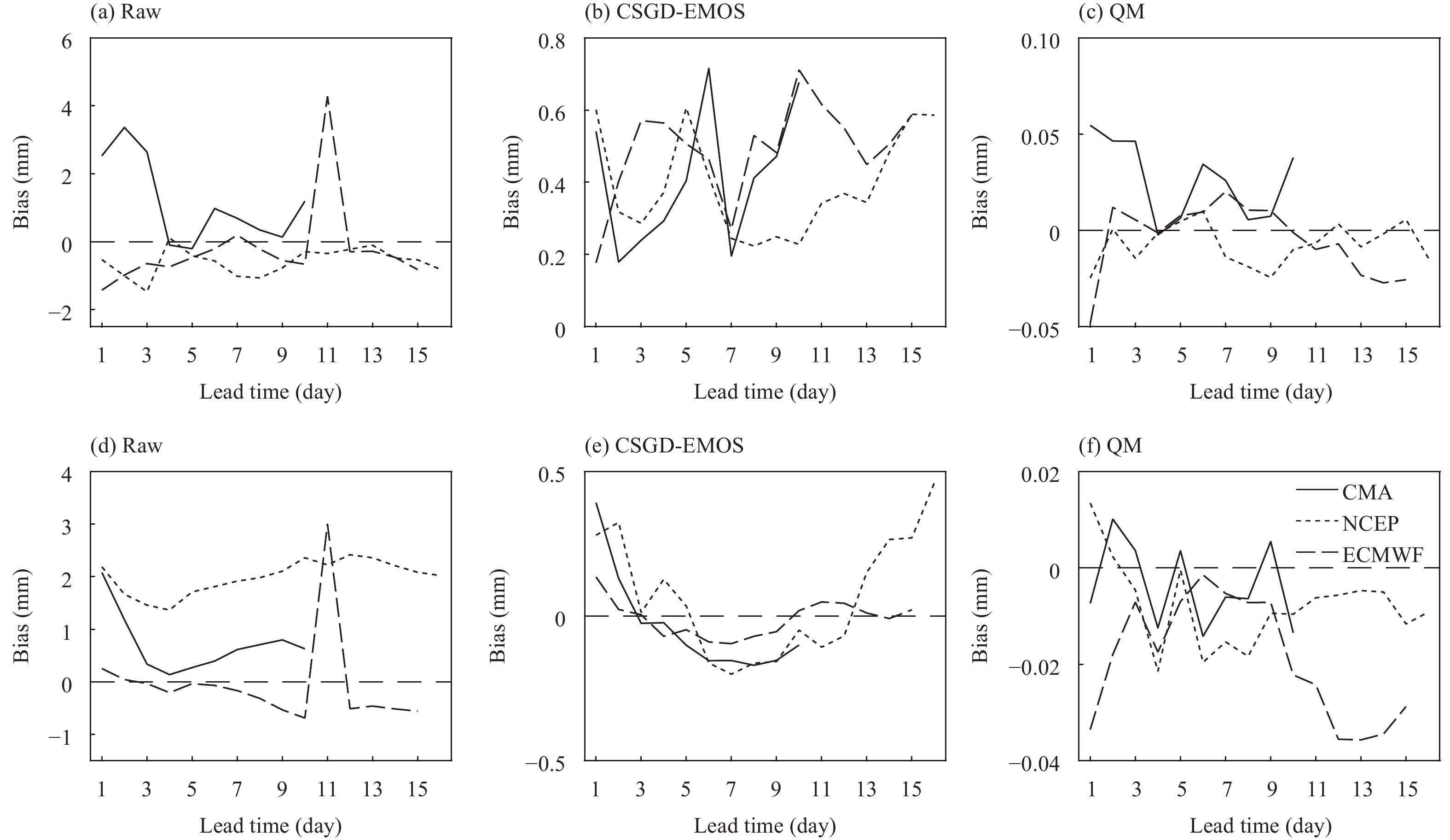Figure 3 Bias for ensemble mean of three EPSs. (a−c) The plum rain season and (d−f) the typhoon season.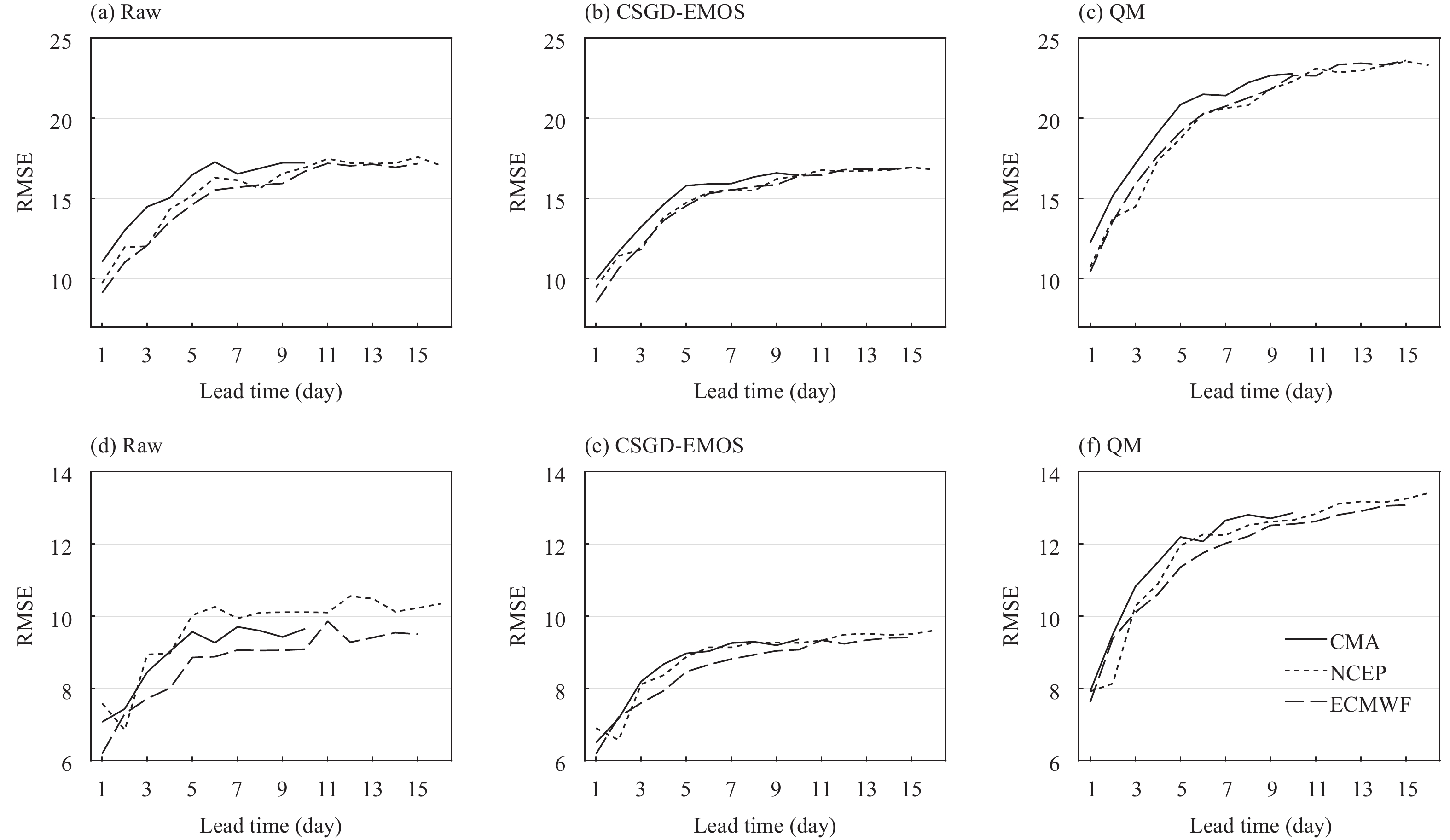Figure 4 As in Fig. 3, but for RMSE.

The Bias metric is significantly improved for the post-processed QPFs, especially by QM. The results from CSGD-EMOS are slightly overestimated, but the effect of this method is still prominent, with Bias < 0.7 mm for all lead times in the plum rain season. In the typhoon season, the post-processed QPFs show a regime in which the forecasts are overestimated for lead times shorter than day + 3 and longer than day + 12. With respect to QM, Bias confined between −0.05 and 0.1 mm can be observed ( Fig. 3c). QM almost reproduces the actual amount of rainfall with an ignorable Bias, particularly in the typhoon season (Fig. 3f).

In terms of the RMSE, a clear deterioration against lead time is found for the raw forecasts. When the lead time extends beyond day + 11, the RMSE becomes stable, indicating that the RMSE becomes insensitive to the lead time. ECMWF is the best EPS in both seasons. QPFs from the CMA have the largest errors in the plum rain season, whereas NCEP has the poorest performance in the typhoon season. It is clear that all the EPSs have a smaller RMSE in the typhoon season.

After post-processing, the improvement in the RMSE is less than that in Bias. The RMSE is a squared metric that gives more weight to heavy rain, and thus, it is apparent that QM behaves unsatisfactorily during heavy rain. Poorer heavy rain forecasts are produced for lead times longer than day + 2. The effect of CSGD-EMOS on heavy rain is positive and the improvement is substantial for the CMA results in the plum rain season. Errors in the NCEP results are markedly reduced by CSGD-EMOS in the typhoon season. Based on Fig. 4, it is clear that the inferior EPSs are improved more significantly.

4.2 Categorical metrics

Figures 513 show the categorical evaluation metrics as a function of the lead time. Figures 57 are the FBI metrics of the raw, CSGD-EMOS, and QM post-processed QPFs, respectively. In brief, the raw FBI in the plum rain season prevails over that in the typhoon season. Over the entire verification period, all the QPFs show a well-marked shift from over- to under-estimation with increasing thresholds, meaning that “false alarm” forecasts dominate in light rain events, whereas more “miss” forecasts take place in heavy rain. In other words, all the EPSs overestimate drizzle, but underestimate heavy rain. This is a well-known phenomenon in many NWP models and has been reported elsewhere (Hamill, 2012; Shrestha et al., 2013). Regardless of the over- or under-estimation, the disagreement between the forecasts and the observations increases with the lead time. The QPFs of NCEP are slightly better in the plum rain season. The QPFs of ECMWF generally lose their advantage in the evaluation of higher thresholds. Based on the performance at the threshold of 20 mm (24 h)−1, all the QPFs gradually fail to accurately hit the occurrence of heavy rain as the lead time increases.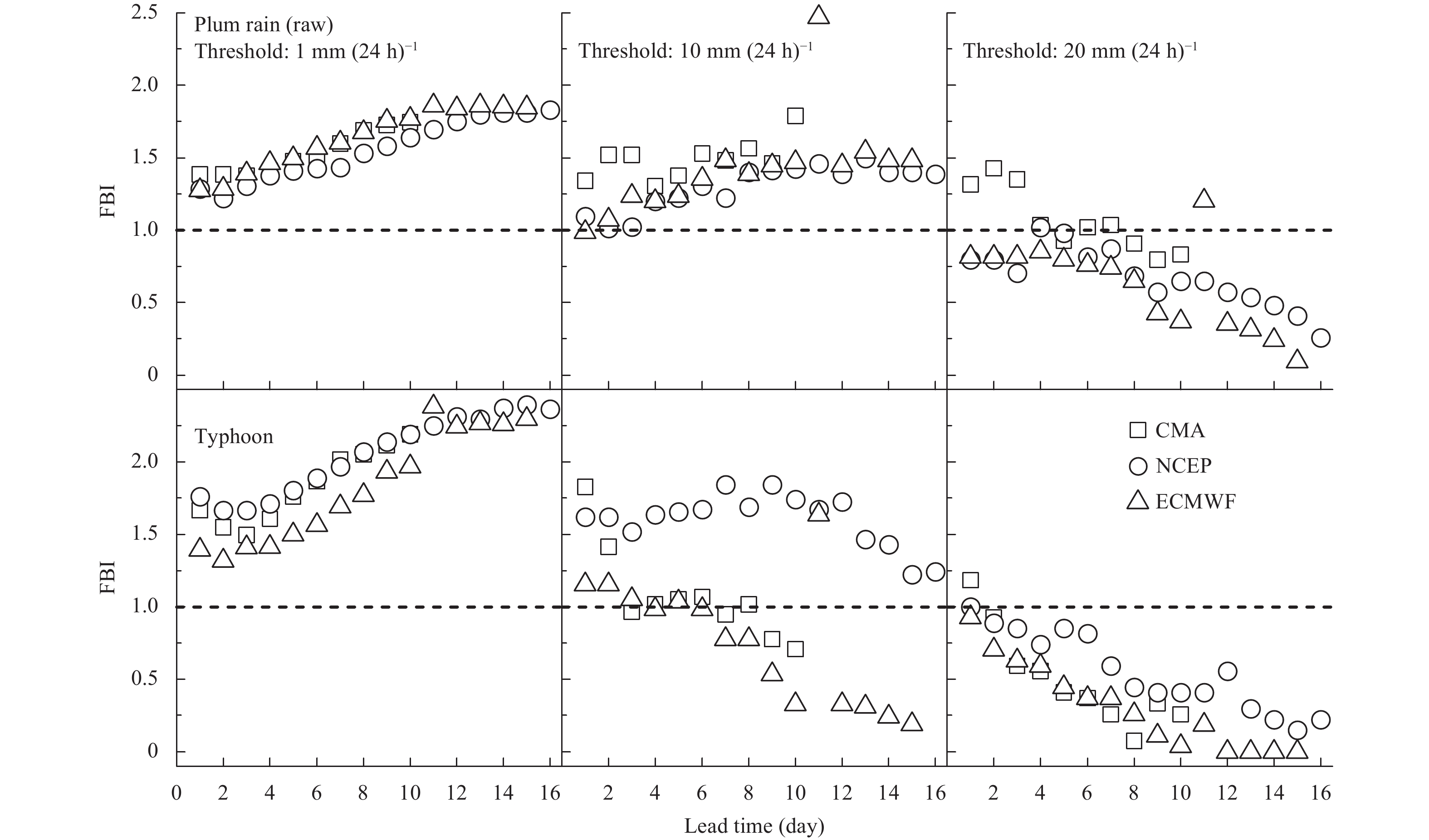Figure 5 FBI of the three EPSs in two seasons computed from the raw QPFs.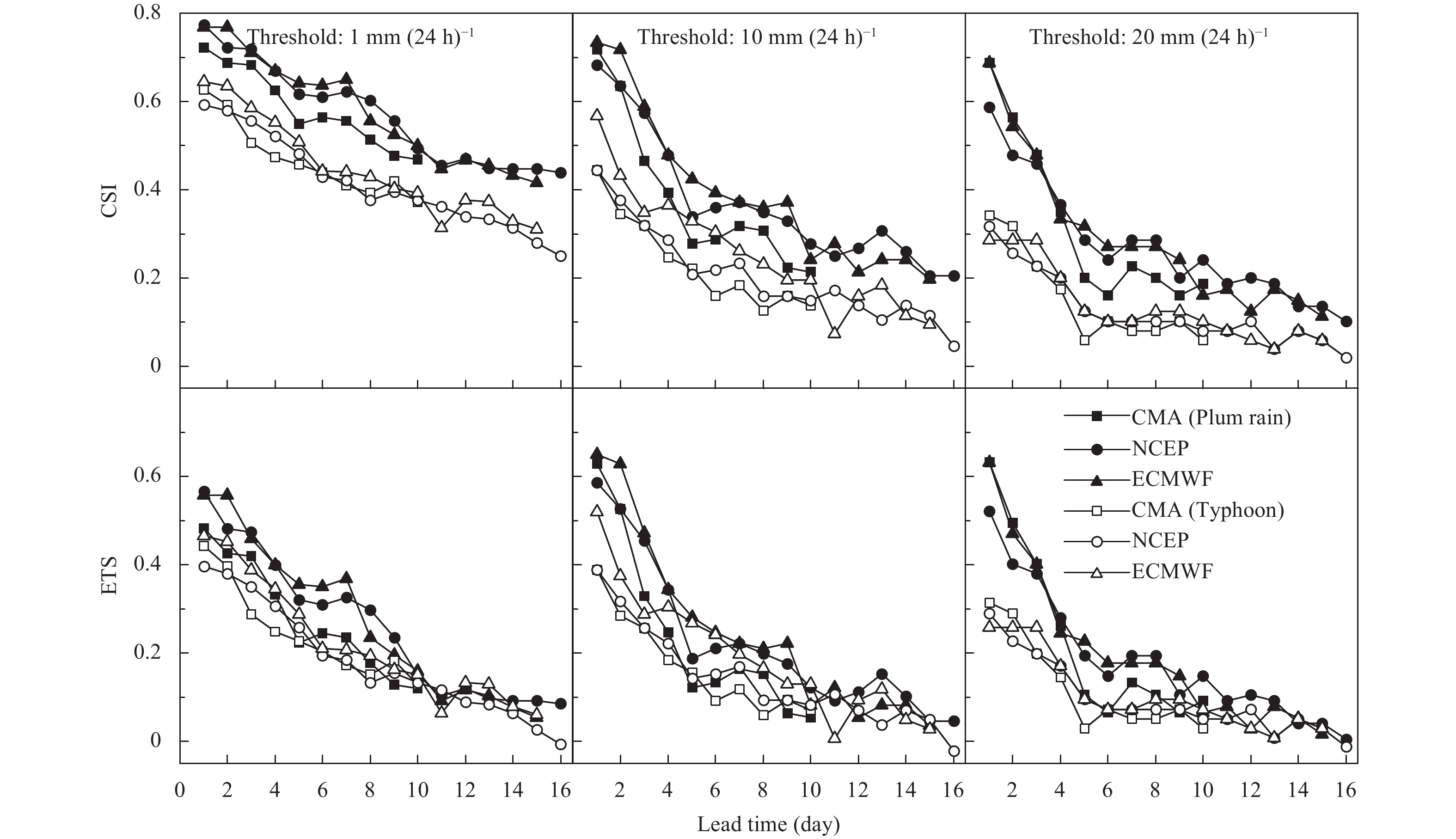Figure 13 CSI and ETS of the three EPSs in two seasons computed from QPFs processed by QM.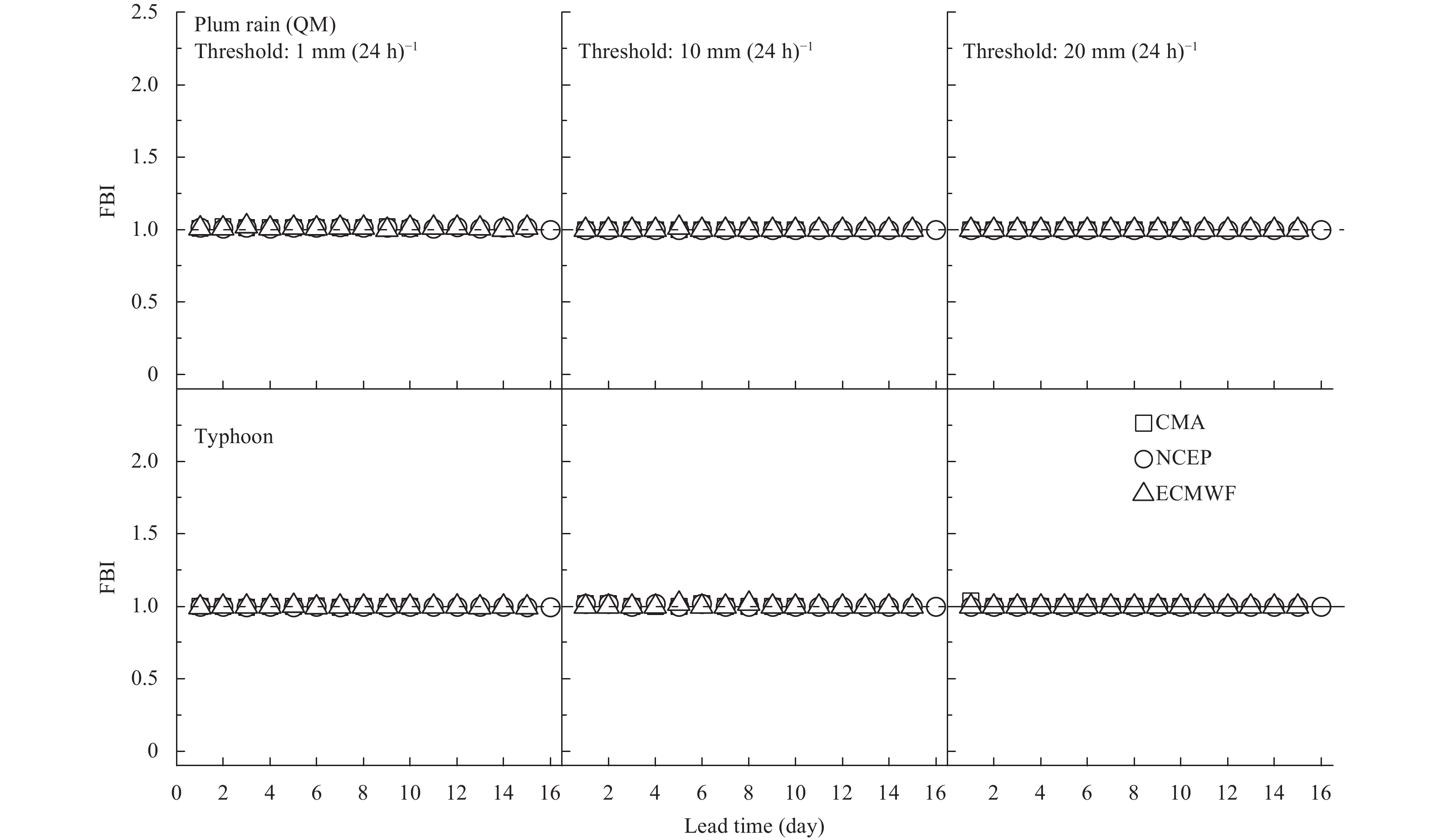Figure 7 FBI of the three EPSs in two seasons computed from the QPFs processed by QM.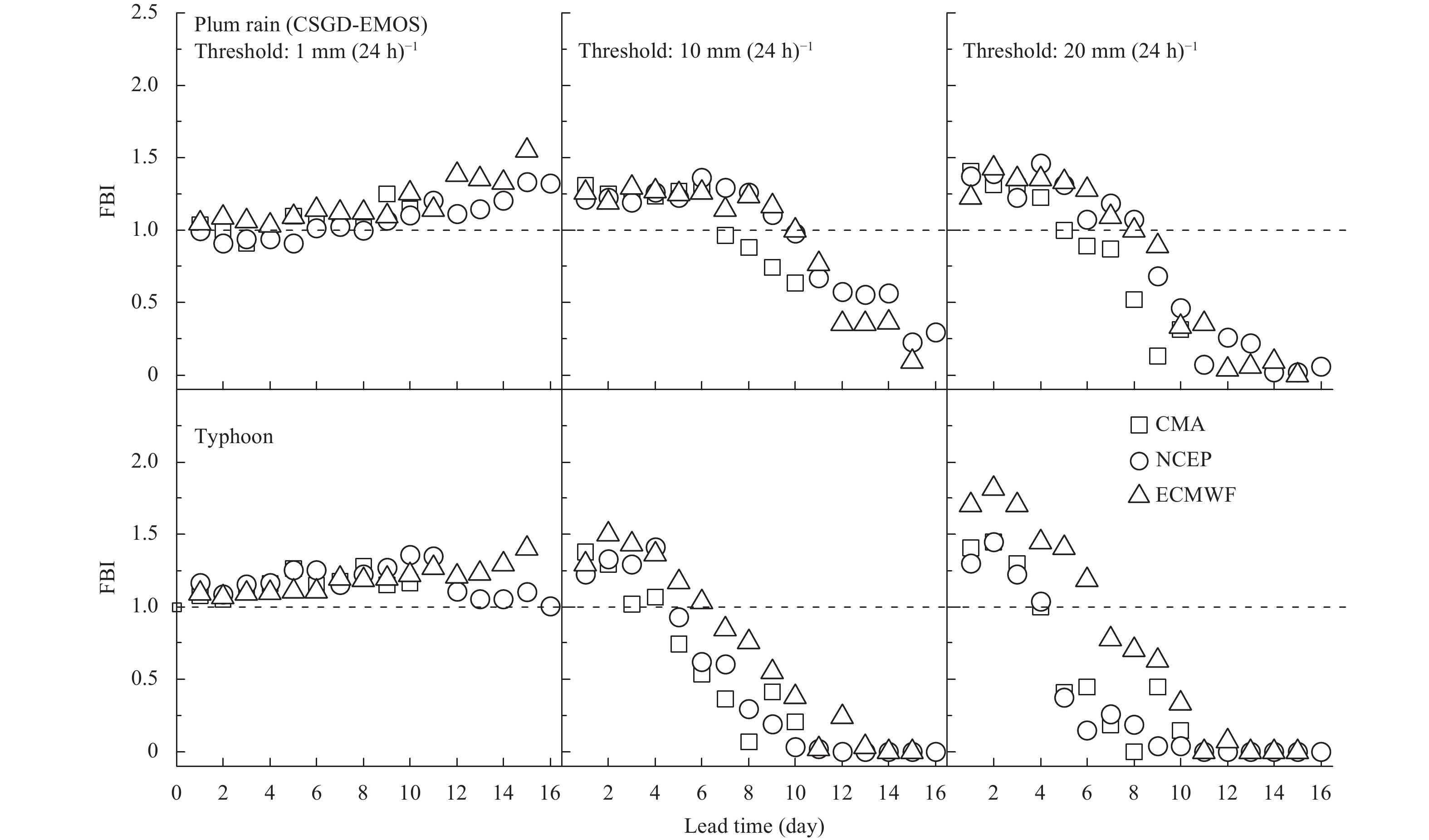Figure 6 FBI of the three EPSs in two seasons computed from the QPFs processed by CSGD-EMOS.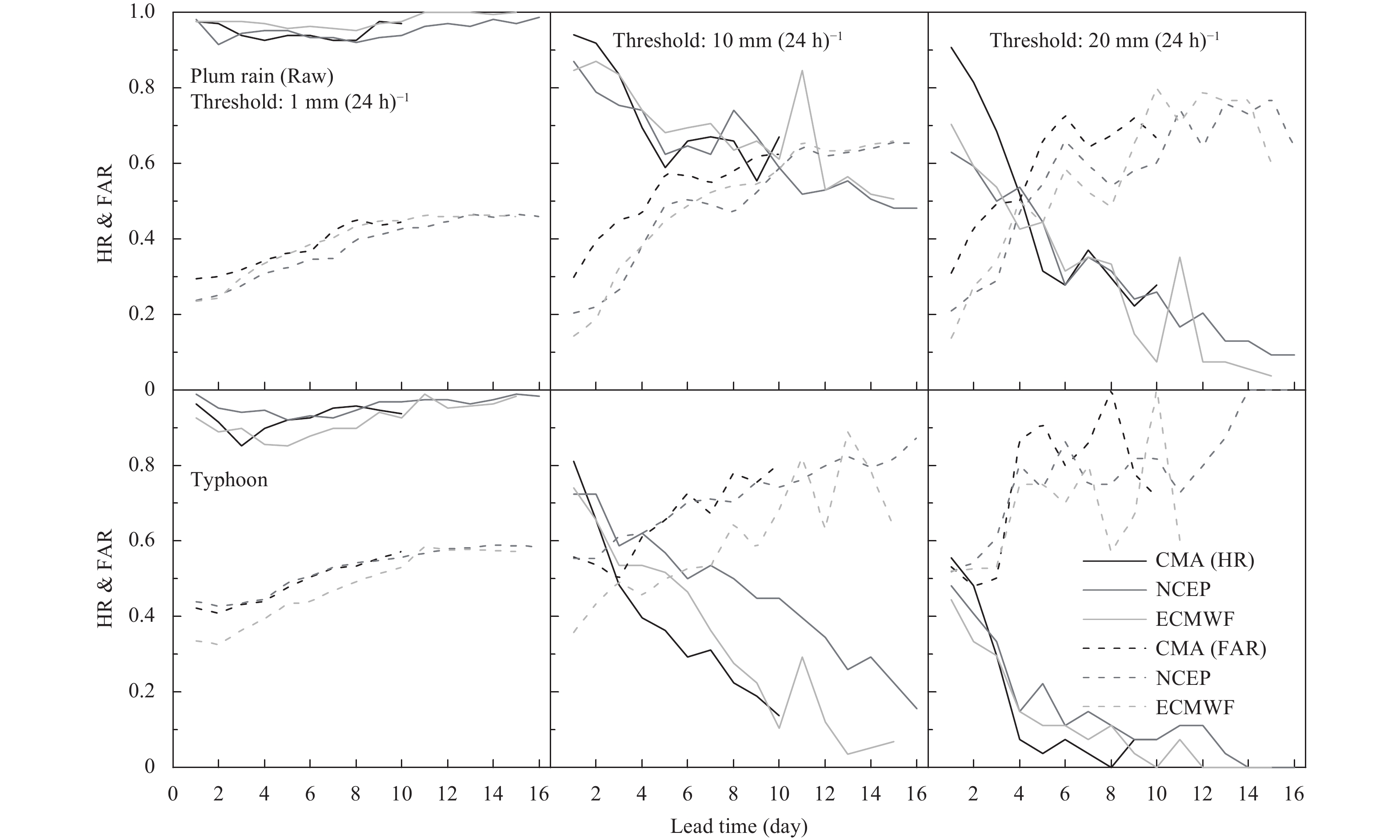Figure 8 HR and FAR of the three EPSs in two seasons computed from raw QPFs.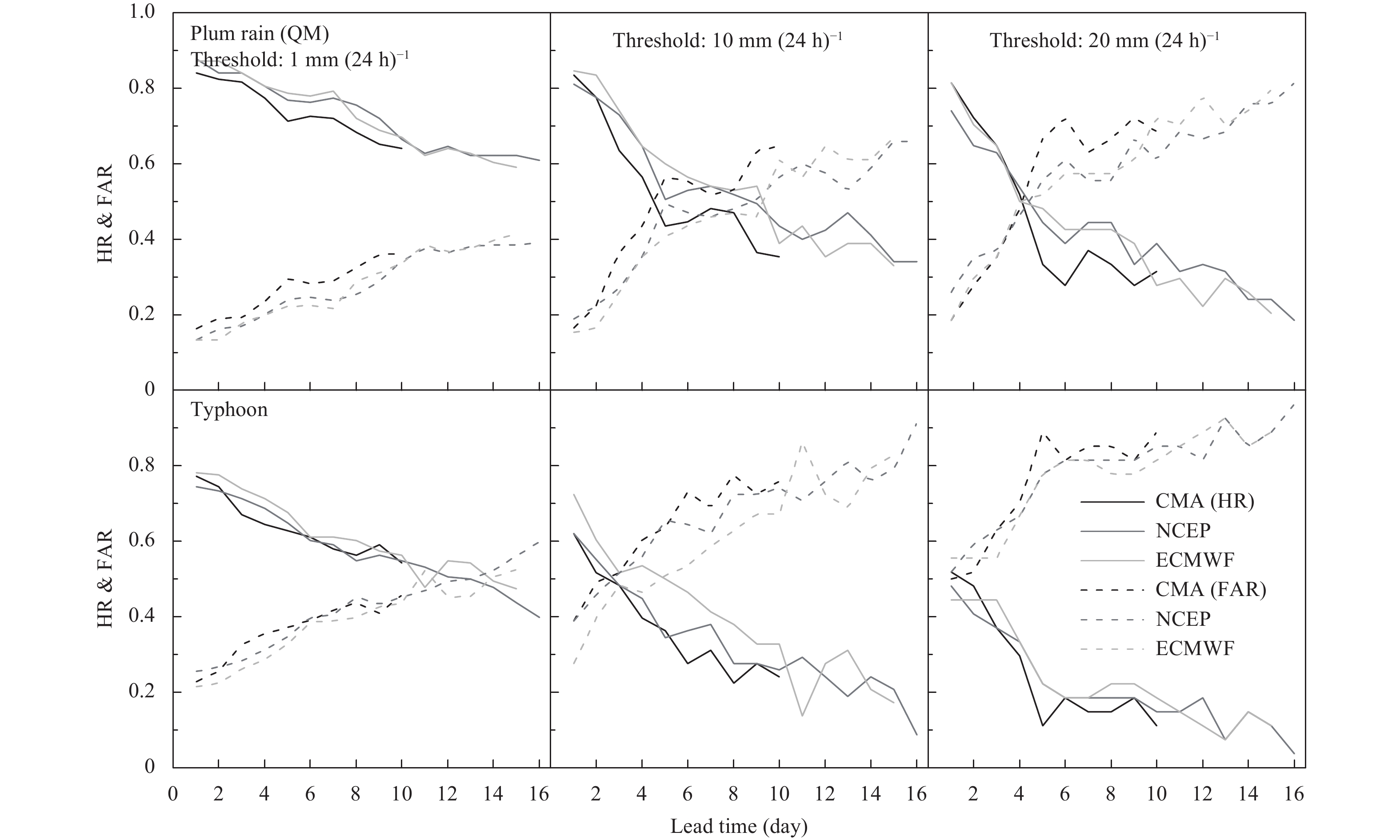Figure 10 HR and FAR of the three EPSs in two seasons computed from QPFs processed by QM.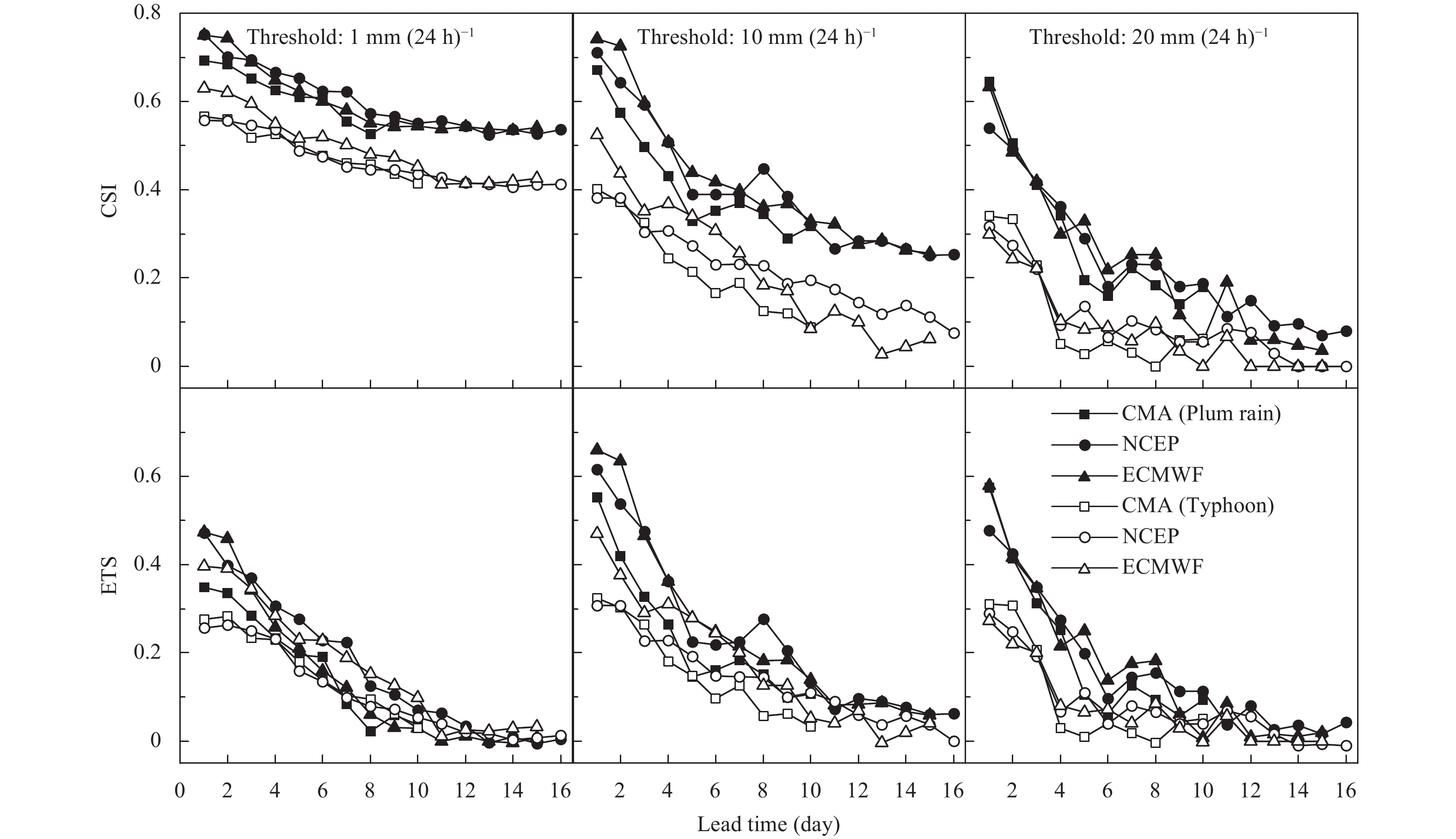Figure 11 CSI and ETS of the three EPSs in two seasons computed from raw QPFs.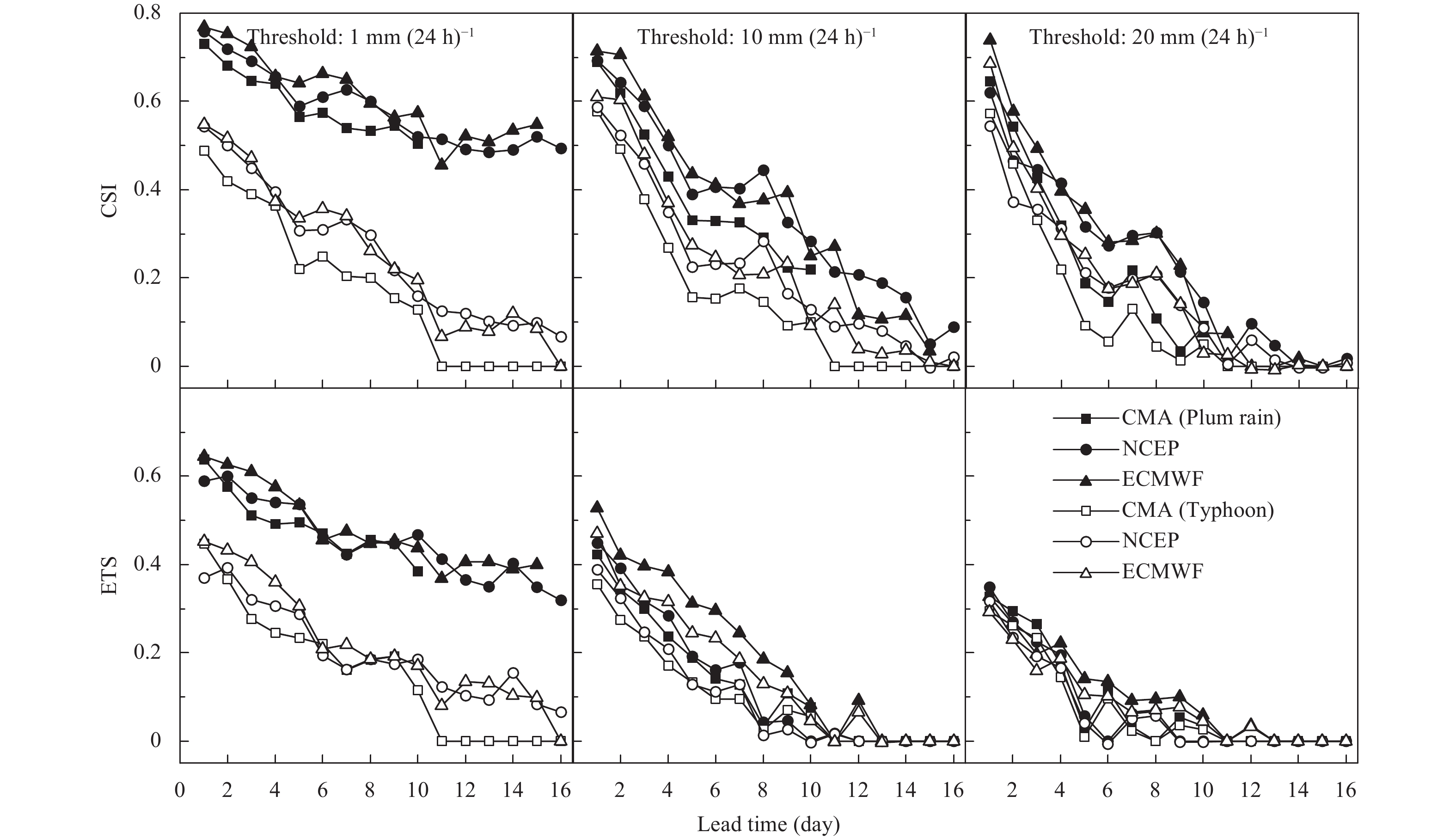Figure 12 CSI and ETS of the three EPSs in two seasons computed from QPFs processed by CSGD-EMOS.

After post-processing, the scores of the FBI are significantly improved and the gain following QM is extraordinarily high. CSGD-EMOS clearly provides better results in light rain events. For heavier rain, unbiased forecasts only last for eight days in the plum rain season and three days in the typhoon season. With increasing lead times, all the EPSs miss the occurrence of moderate and heavy rain and the FBI decreases to zero (Fig. 6). Based on Fig. 7, it is clear that the results from QM can be regarded as unbiased over all lead times. It is difficult to distinguish which EPS is better after post-processing.

Figures 810 compare HR and FAR. As expected, except for raw light rain, a decreasing HR is always accompanied by an increasing FAR, indicating that both HR and FAR deteriorate with increasing lead times. When the given threshold is lower, for example, 1 mm (24 h)−1, HR is consistently high for all lead times. All the QPFs have HR > 0.9 (left-hand panel in Fig. 8), which may be attributed to the fact that most members tend to predict slightly more rain regardless of whether it actually occurred, so that the number of “hits” increases by chance. This is also why FAR is fairly high at the start. Overall, both the raw HR and FAR are more desirable in the plum rain season and at a lower threshold of precipitation. All the QPFs provide skillful forecasts for at least day + 8.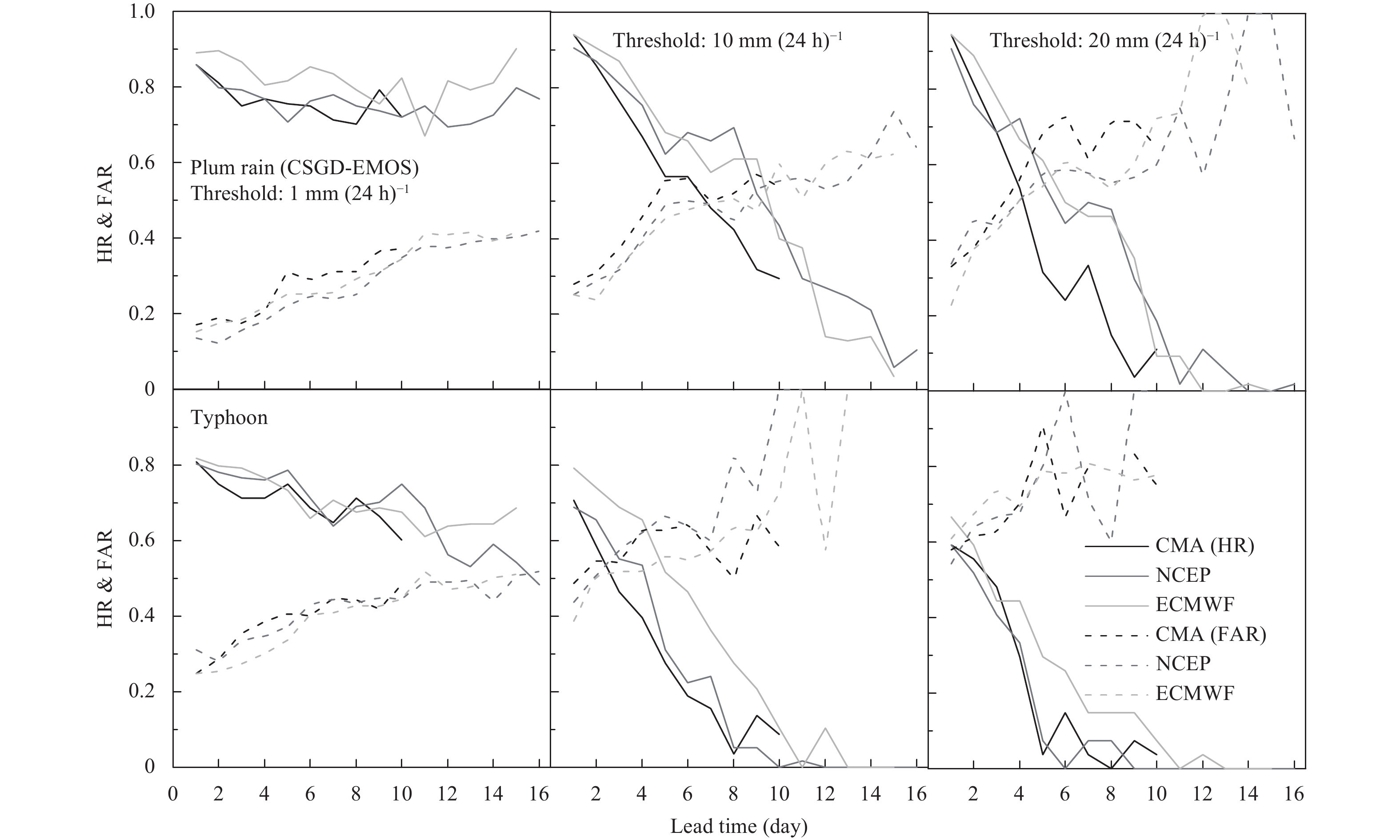Figure 9 HR and FAR of the three EPSs in two seasons computed from QPFs processed by CSGD-EMOS.

With respect to post-processing, neither method has as encouraging effects as before. The post-processed HR for light precipitation is not so good as the raw case. The main contributor to the post-processing can be regarded as an improvement in longer lead times. CSGD-EMOS slightly outperforms QM in light rain forecasts, with a generally higher HR and lower FAR. QM can increase the forecast skill by an equivalent of up to five days of additional lead time for heavy rain. Consistent with the raw forecasts, ECMWF and NCEP provide more preferable results than CMA. Coupled with FBI, some interesting information can be found. First, the more unbiased the FBI is, the more symmetrically the graph is divided by HR = FAR = 0.5 axes. This is due to the existing relation of selected metrics: FBI = HR/(1 − FAR) (Su et al., 2014). Thus, if the HR and FAR scores tend to be symmetrical with an axis of symmetry greater (less) than 0.5, the conclusion is that the FBI is greater (less) than 1. Second, it seems that the unbiased forecasts by QM are caused by the equal number of “miss” and “false alarm” forecasts. The number of “hit” forecasts is not substantially increased by QM. For precipitation > 10 mm (24 h) −1, an even lower HR is obtained, indicating that some “hit” forecasts in raw QPFs are changed into a “miss” or “false alarm” to achieve unbiased forecasts.

The raw CSI and ETS in Fig. 11 show extra explanations for the extremely high HR for light rain. When the relatively high CSI excludes the correct forecasts by chance (r), the score (ETS) decreases visibly, roughly half the CSI, proving that majority of the correct forecasts contributing to high CSI scores are derived from random chance. Both CSI and ETS deteriorate with lead time. The discrimination in ETS between two seasons is not so remarkable as CSI. Skillful forecasts can extend to 8 days ahead. The function of CSGD-EMOS and QM can be regarded as an entire promotion in skills, particularly for ETS in the lowest threshold (Figs. 12, 13). Consistently, QM outperforms CSGD-EMOS. After post-processing, the skillful forecasts are extended beyond 13 days in advance.

4.3 Probabilistic metrics

Figures 1416 present the BSS and reliability diagram for the QPFs from three EPSs as a function of the forecast lead times. Based on the BSS (Fig. 14), the raw forecasts already show fairly high skills compared with the climatology, with lead times of up to 10 days, except for NCEP in the typhoon season. In general, a higher BSS is obtained in the plum rain season than in the typhoon season, especially for shorter lead times. The BSS deteriorates sharply with an increase in lead time. This probabilistic skill score is marginally ameliorated with increasing thresholds, showing an encouraging performance in heavier rain events. The QPFs from ECMWF occupy the first place, as reported by Hamill (2012), followed by the CMA. The QPFs from NCEP show surprisingly poor skills in the typhoon season with a BSS < 0 for most lead times. There is a clear V-shape in the BSS at lead times of + 11 days for ECMWF raw forecasts. This is caused by the same reason as for the Bias metric ( Fig. 3), namely, the different resolutions in the first 10 days (about 0.28°) from the last five days (about 0.56°).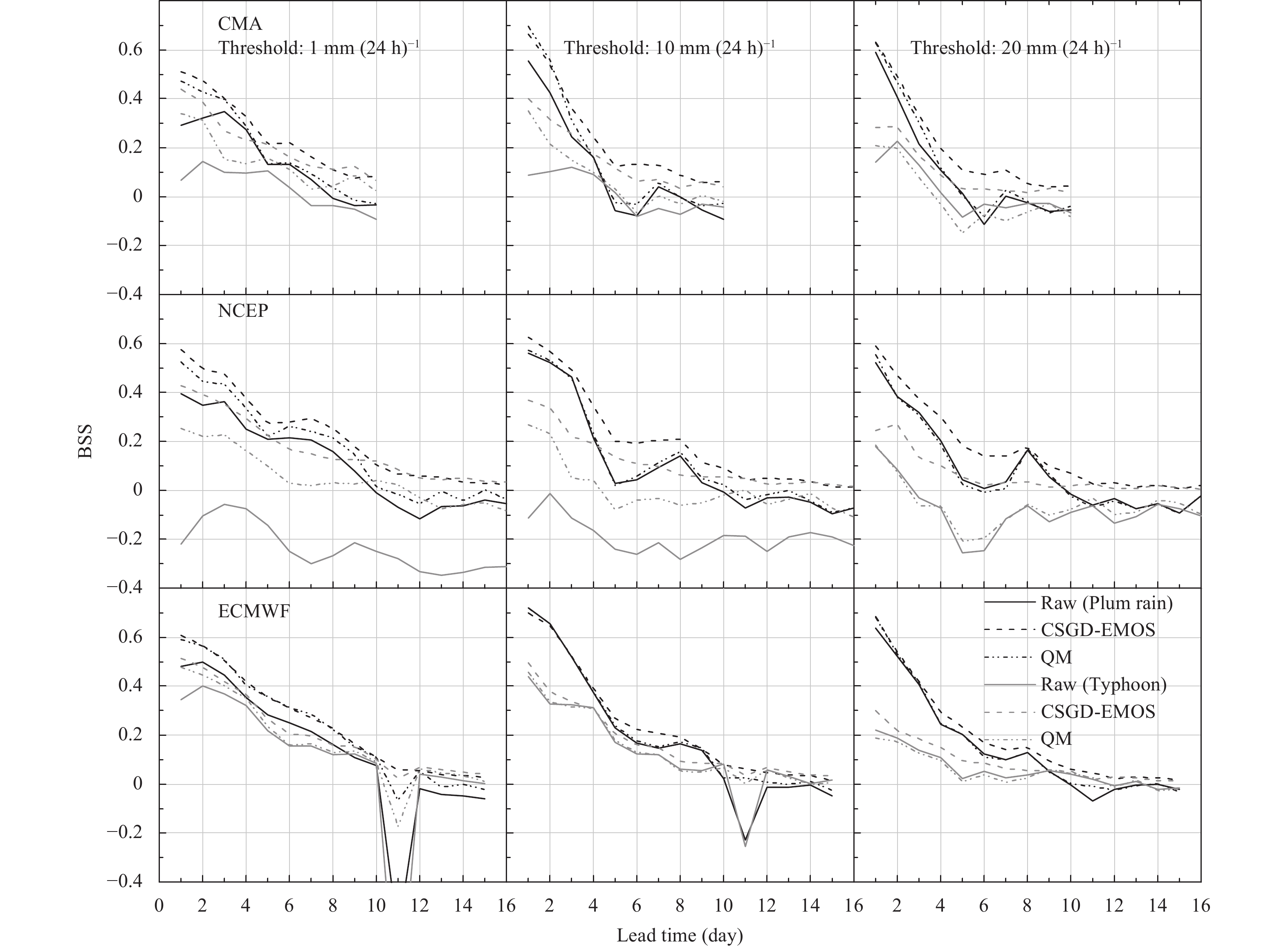Figure 14 BSS of three EPSs in two seasons computed from the QPFs. Black lines are the results from the plum rain season and the results from the typhoon season are shown in gray. The solid lines are the BSS for raw QPFs. The dashed lines indicate the BSS for CSGD-EMOS and the BSS for QM is indicated by dash-dot lines.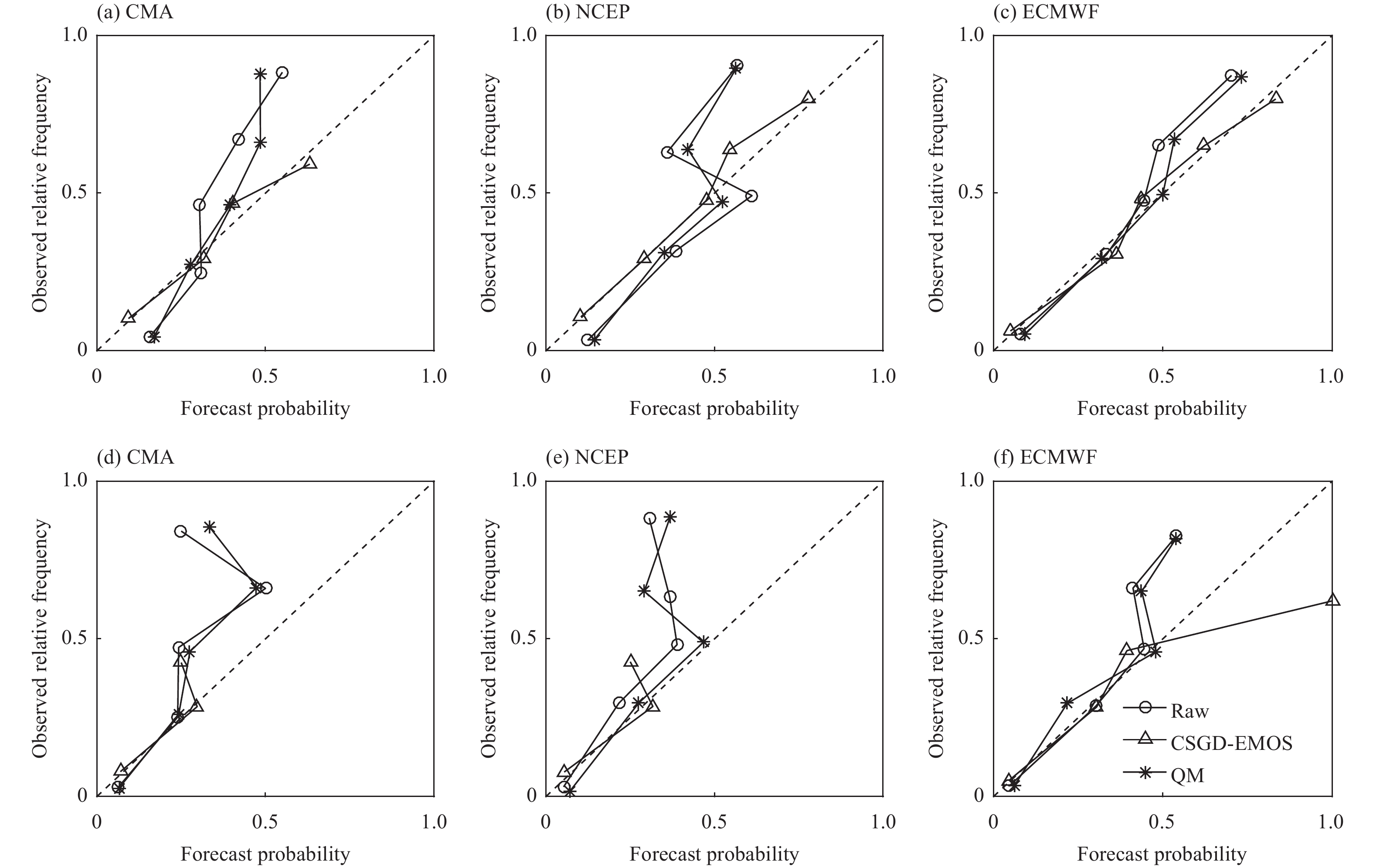Figure 16 Reliability diagram of the three EPSs in two seasons for a threshold of 10 mm at a lead time of + 5 days. The upper panels are results for the plum rain season and the lower panels are for the typhoon seasons.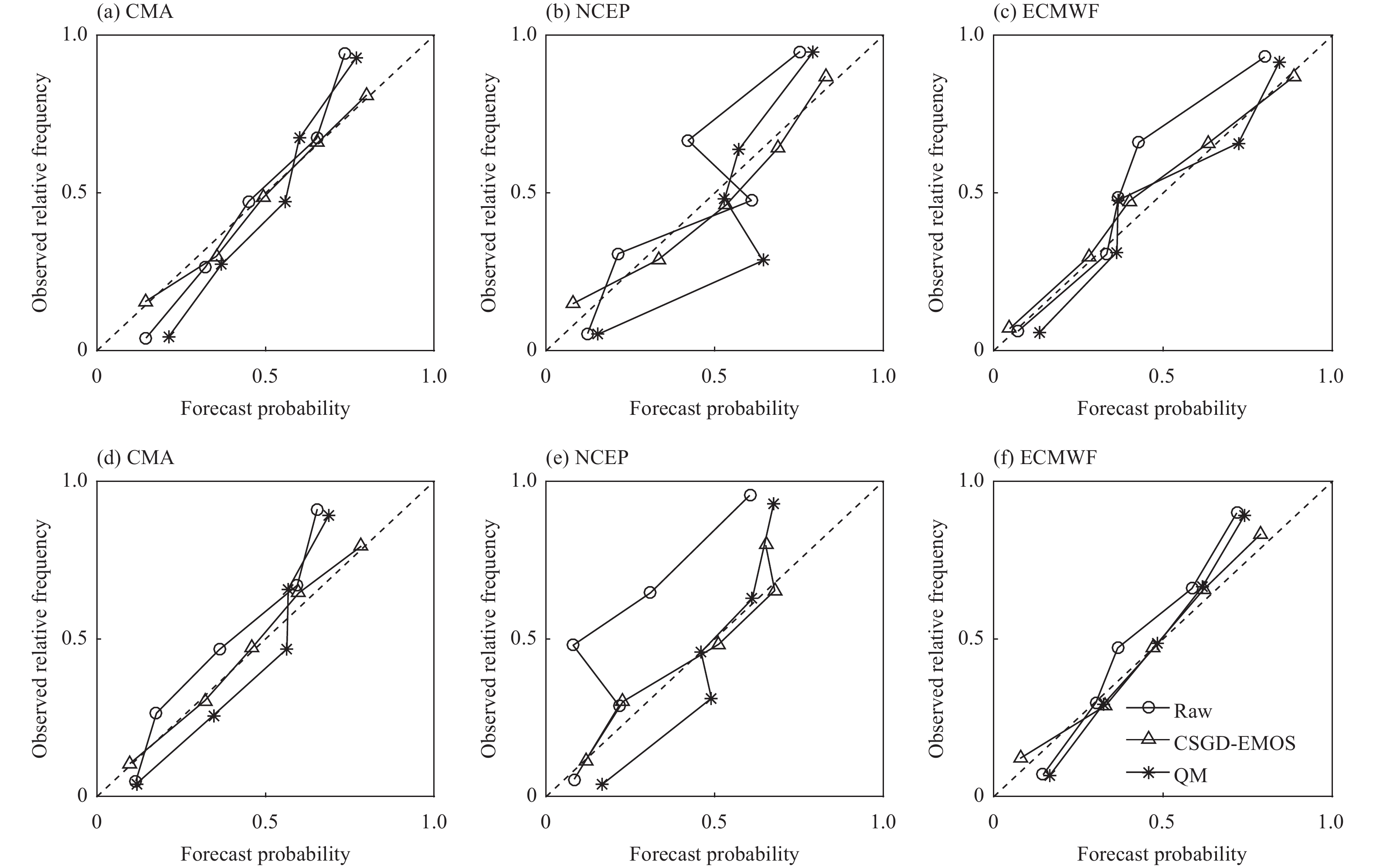Figure 15 Reliability diagram of the three EPSs in two seasons for a threshold of 1 mm at a lead time of + 5 days. The upper panels are the results for the plum rain season and the lower panels are for the typhoon season.

It is not difficult to detect that the improvements are significant after post-processing, especially for the CMA and NCEP. The skillful forecasts in the typhoon season by the NCEP are increased up to a lead time of 10 days and the ECMWF gives better QPFs in light rain. The CSGD-EMOS behaves much better than QM. A smoother change with lead times is provided by the CSGD-EMOS. For the CMA, the CSGD-EMOS outperforms QM by an additional + 1 day. The difference between the CSGD-EMOS and QM decreases with increasing lead times.

Figures 15 and 16 show reliability diagrams for thresholds of 1 and 10 mm (24 h)−1 at a lead time of + 5 days. Most EPSs miss the forecasts for heavy rain events and the related evaluation is therefore omitted. For the raw forecasts, more reliable forecasts are obtained by the ECMWF and CMA. Forecasts in the plum rain season have a higher reliability than those in the typhoon season. An apparent underestimation exists in the NCEP forecasts. After post-processing, similar to the BSS, a higher reliability is provided by CSGD-EMOS and the advantage of this method becomes clearer for heavier rain events and longer lead times (omitted here). QM does not improve the reliability of forecasts and tends to overestimate forecasts for lower frequency events, but underestimates forecasts for higher frequency events, which can be seen from the cross-diagonal curve shown in Figs. 15, 16.

4.4 Overall metrics

Figure 17a clearly shows that for most lead times, the post-processed QPFs are inferior to the raw forecasts. In general, QM is slightly better than CSGD-EMOS. Encouraging improvement occurs for the CMA results, especially for shorter lead times. It seems that CSGD-EMOS has a negative effect on the QPFs from the NCEP. In the typhoon season (Fig. 17b), the post-processing methods show a positive effect for the QPFs, especially the NCEP. An impressive improvement can be seen for both CSGD-EMOS and QM for longer lead times.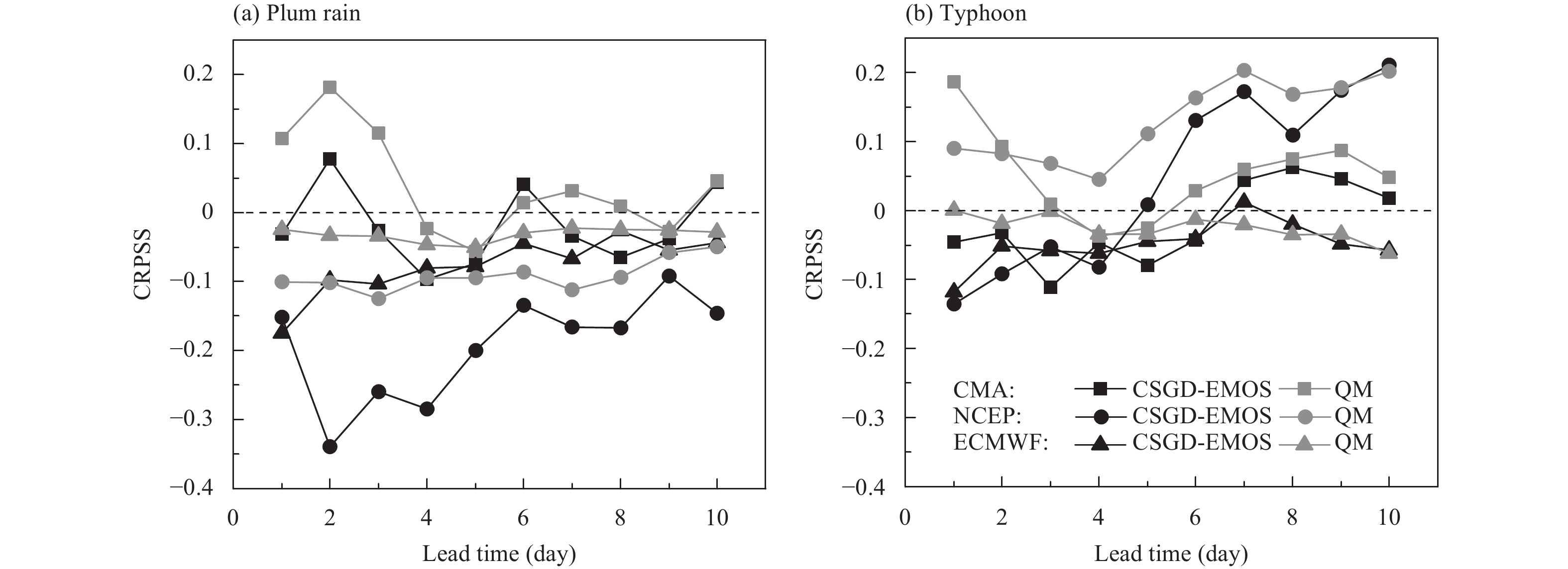Figure 17 CRPSS of the three EPSs in two seasons computed from QPFs. (a) Plum rain season and (b) typhoon season.
5 Conclusions and discussion

We conducted a comprehensive evaluation of ensemble precipitation from three operational global EPSs during the plum rain and typhoon seasons from 2009 to 2013 in the Qu River basin, eastern China. We compared different precipitation thresholds, seasons, and post-processing methods. The results show that most of the raw QPFs can be useful inputs for hydrological models with a lead time of 8–10 days ahead and the post-processing methods are able to improve the skill of forecasts and extend the effective lead time beyond 10 days.

All the verification metrics are characterized, with pronounced discriminations between the plum rain and typhoon seasons, indicating that it is necessary to assess the forecasts by season. In general, except for the bias and RMSE, the raw forecasts in the plum rain season are always superior to those in the typhoon season based on either the categorical or probabilistic metrics. This can be explained by the diverse rainfall patterns dominating in these two seasons and the variation in the capacity of the EPSs to forecast different types of rainfall. Previous studies have shown that NWPs model large-scale synoptic systems better as a result of their relatively slow evolution (Robertson et al., 2013), whereas the rainfall from convective systems is less well predicted because the processes involved evolve rapidly and generally occur on spatial scales finer than the resolution of the NWP model (Shrestha et al., 2013). The rainfall in the plum rain season, as consequence of the quasi-stationary front, stays in a relatively evolution-free state for a long time and takes place over a wide spatial scale compared with that in the typhoon season. This results in more reliable QPFs by the EPSs.

Two statistical post-processing methods were applied to the raw QPFs from the TIGGE archives and the effects varied with the lead time, selected verification metrics, precipitation thresholds, and EPSs. This is consistent with the conclusion of Wilks and Hamill (2007) that no single post-processing technique is optimum for all applications. In addition, the effects of post-processing are also sensitive to the choice of metrics. Verkade et al. (2013) found that if the objective functions used to estimate the parameters of the post-processing technique are similar to the verification metrics used, then this particular technique scores well in terms of that metric. The CSGD-EMOS is a more skillful post-processing method in terms of probabilistic skill, according to the BSS and reliability diagram. QM seems to lose its advantage in probabilistic metrics, but performs fairly well according to Bias and most of the categorical metrics. This conclusion is consistent with the results of Zhao et al. (2017). Madadgar et al. (2014) verified that QM usually functions well in cases where the CDFs of the observed and modeled data are distant or the probability density functions do not overlap. However, the CDFs in this study, on some occasions, are both close and crossed, which does not allow QM to reach its full potential.

As for the poor performance in forecasting heavy rains in both post-processing methods (CSGD-EMOS is better than QM according to the RMSE), it is probably helpful to use other probability density functions. Hemri et al. (2014) and Scheuerer (2014) introduced an adaptation of the generalized extreme value distribution to post-processing QPFs and showed a good performance in heavy rain. They also suggested that the incorporation of ensemble forecasts in the neighborhood of the predictive distribution benefits the forecast at high quantiles. Moreover, according to other studies (Hamill et al., 2008; Swinbank et al., 2016), the accuracy and reliability of forecasts after post-processing will depend on the amount of data available, particularly for heavy rain.

The diverse performance at different precipitation thresholds implies that EPSs have varying degrees of ability to capture rainfall of different magnitudes. As reported in previous work (Hamill, 2012; Shrestha et al., 2013), EPSs behave much less successfully in either light or heavy rain. There is a tendency to predict too high a frequency of light rain using the FBI, HR, and FAR metrics and rain > 20 mm (24 h) −1 is not sufficiently produced. Fortunately, post-processing affects both these under- or overestimations to some degree (Fig. 3). Processing the data, both modeled and observed, as areal precipitation in this study limits the assessment of the spatial variation in the skill of precipitation forecasts of the TIGGE data. Simply averaging the data as areal precipitation may result in the elimination of some local extreme values. This kind of influence may be more remarkable in the typhoon season as typhoons only affect a small part of the basin.

The comparison among EPSs shows that the QPFs of the CMA behave less satisfactorily in terms of Bias, RMSE, and some categorical metrics. The QPFs of the NCEP and ECMWF present comparable skills, although those of the ECMWF are more satisfactory in the typhoon season and those of the NCEP are more satisfactory in the plum rain season. The ECMWF ensemble is known to have the most desirable performance in predicting the position, intensity, and propagation speed of cyclones (Swinbank et al., 2016). This is why the ECMWF usually behaves better than the others in the typhoon season when cyclone precipitation dominates. In general, the less skillful the raw forecasts are, the more improvements are obtained by post-processing.

This study indicates that the effective lead time of using TIGGE dataset as a useful input to hydrological models varies with the season, threshold, and verification metrics. All the EPSs provide skillful raw forecasts with lead times of 8–10 days. There are limited cases when the forecasts are only useful for shorter lead times. For instance, the forecasts by the NCEP in the typhoon season are not useful at any lead time. Whether the forecasts are skillful or unskillful also depends on the verification metrics. This study shows that the TIGGE dataset provides skillful forecasts with fairly long lead times for flood forecasting based on most of the metrics.

In conclusion, TIGGE precipitation, in spite of some errors, is suitable for use in medium-term precipitation or flood forecasting. This study evaluated different EPSs and no large ensemble for multiple centers was considered. Based on previous studies (Liu and Xie, 2014; Hamill and Scheuerer, 2018), an ensemble of multiple EPSs outperforms a single EPS and a large ensemble based on appropriate combination methods (e.g., Bayesian model averaging) will be conducted in our future work.

Acknowledgments. The National Climate Center of the China Meteorological Administration is acknowledged for providing meteorological data for the Qu River basin. Precipitation data were obtained from the ECMWF’s TIGGE data portal. Thanks are given to the ECMWF for development of this portal software and for archives of this immense dataset. We also thank Mr. Scheuerer, the developer of CSGD-EMOS, for sharing his code in Github.

References